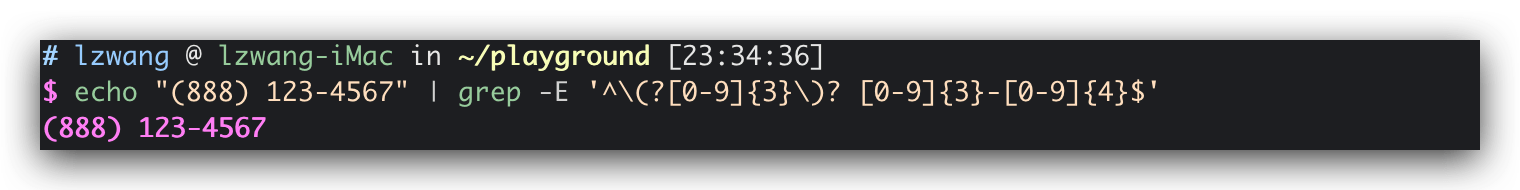## 1. 文本搜索工具`grep`

`grep`接受的参数如下：

-i –ignore-case 忽略大小写
-v –invert-match 不匹配
-c –count 输出匹配到项的数目
-l –files-with-matches 输出匹配项文件名
-L –files-without-matches 输出不包含匹配项的文件名
-n –line-number 在每个匹配行前面加上该行在文件内的行号
-h –no-filename 在同时搜索多个文件时，不输出文件名

## 2. 创建playground进行测试搜索

### 2.1 创建若干文本文件用于测试

 ``````1 2 3 4 5 `````` ``````mkdir ~/playground && cd ~/playground ls /bin > dirlist-bin.txt ls /usr/bin > dirlist-usr-bin.txt ls /sbin > dirlist-sbin.txt ls /usr/sbin > dirlist-usr-sbin.txt ``````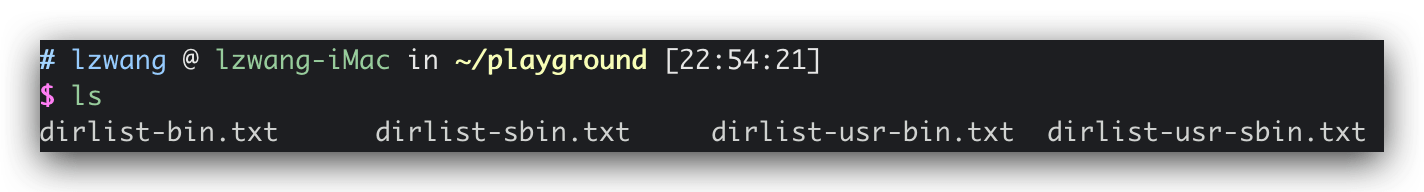### 2.2 简单的搜索测试

2.2.1 在所有以`dirlist`开头的`txt`文件中，搜索`bzip`字段

 ``````1 `````` ``````grep bzip dirlist*.txt ``````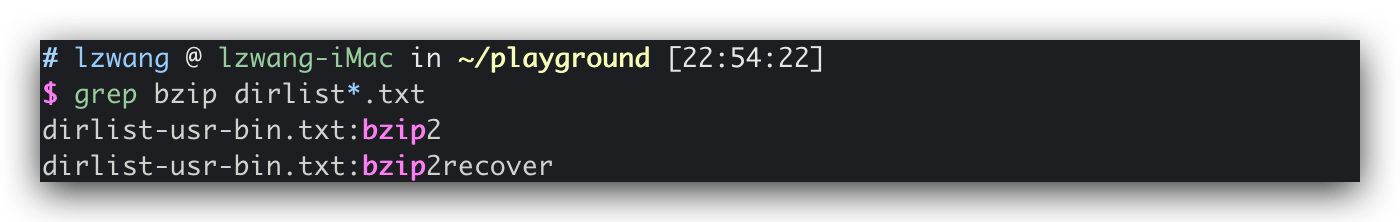2.2.2 输出包含`bzip`字段的文件

 ``````1 `````` ``````grep -l bzip dirlist*.txt ``````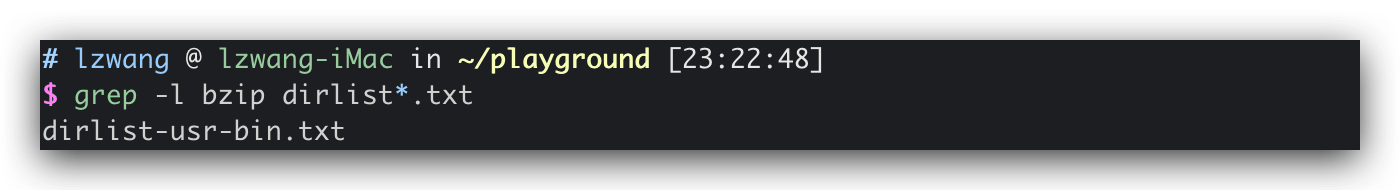2.2.3 输出不包含`bzip`字段的文件

 ``````1 `````` ``````grep -L bzip dirlist*.txt ``````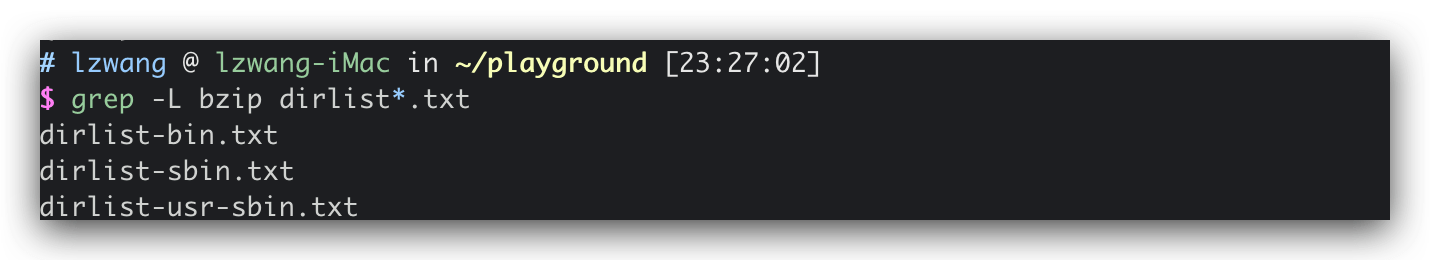## 3. 正则表达式中的元字符

RE中的元字符如下：

`^`, `\$`, `.`, `*`, `?`, `\`, `|`, `-`, `+`, `[`, `]`, `{`, `}`, `(`, `)`

### 3.1 任意字符`.`

 ``````1 `````` ``````grep -h '.zip' dirlist*.txt ``````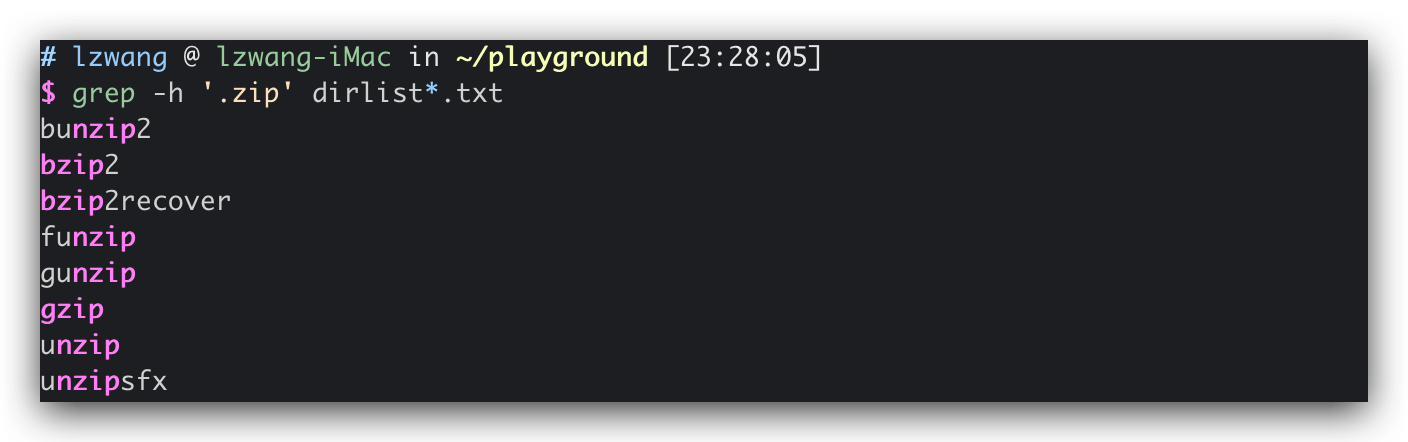### 3.2 锚（开头`^` 结尾`\$`）

 ``````1 2 3 `````` ``````grep -h '^zip' dirlist*.txt grep -h 'zip\$' dirlist*.txt grep -h '^zip\$' dirlist*.txt ``````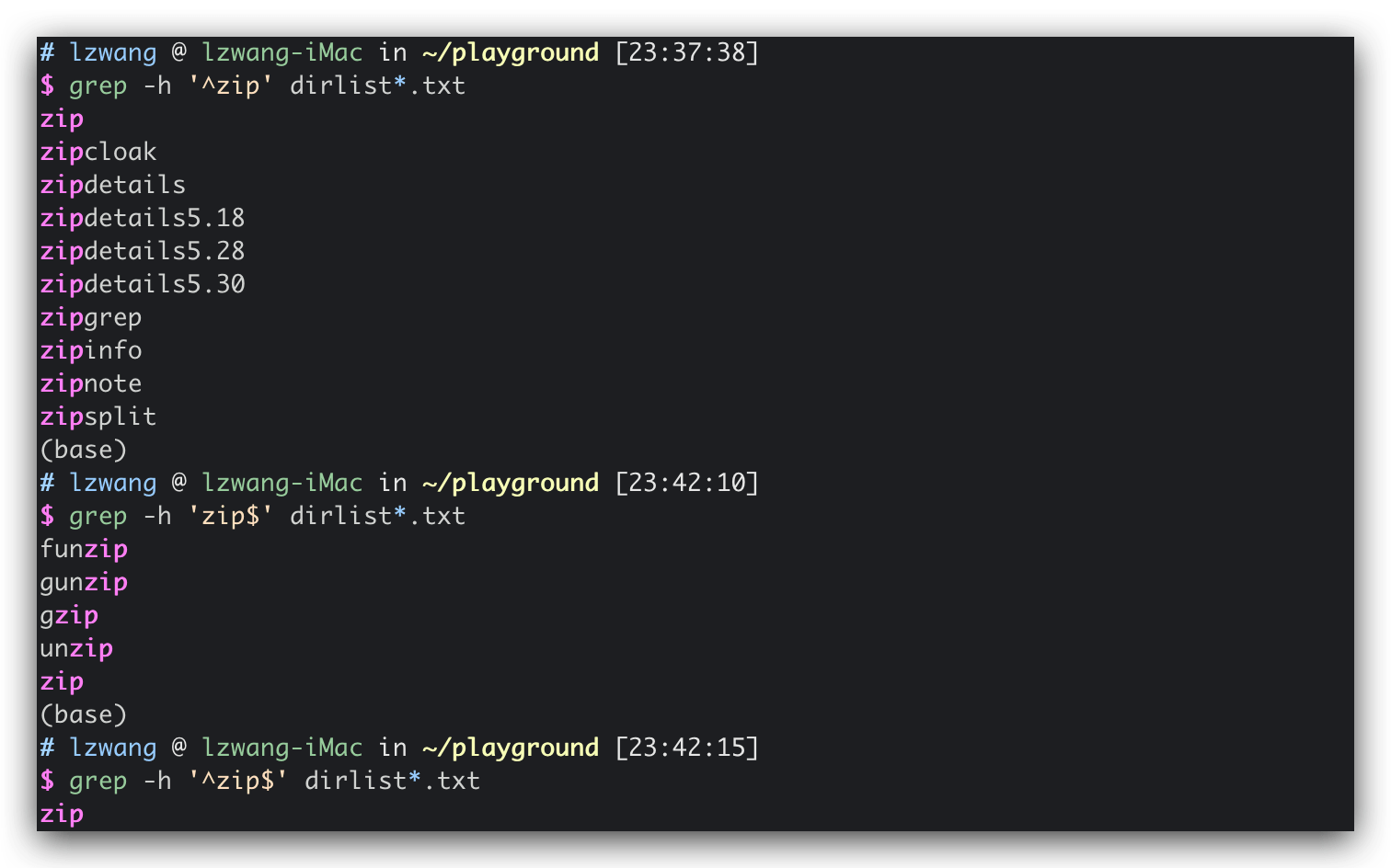### 3.3 匹配字符`[ ]`

3.3.1 特定位置字符匹配

 ``````1 `````` ``````grep -h '[bg]zip' dirlist*.txt ``````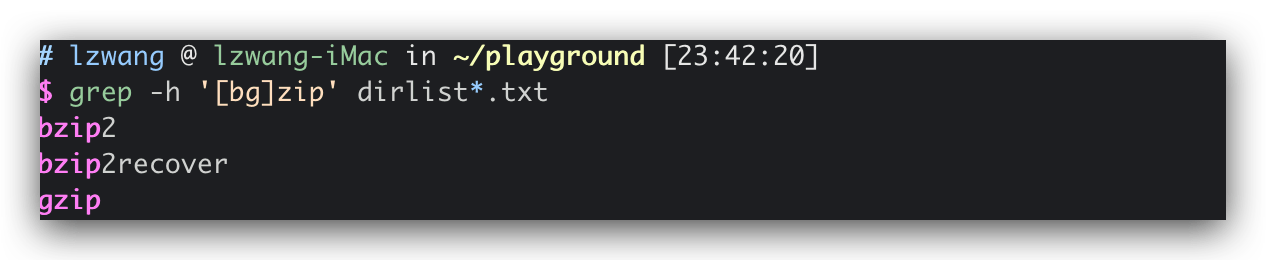3.3.2 特定位置否定匹配

 ``````1 `````` ``````grep -h '[^bg]zip' dirlist*.txt ``````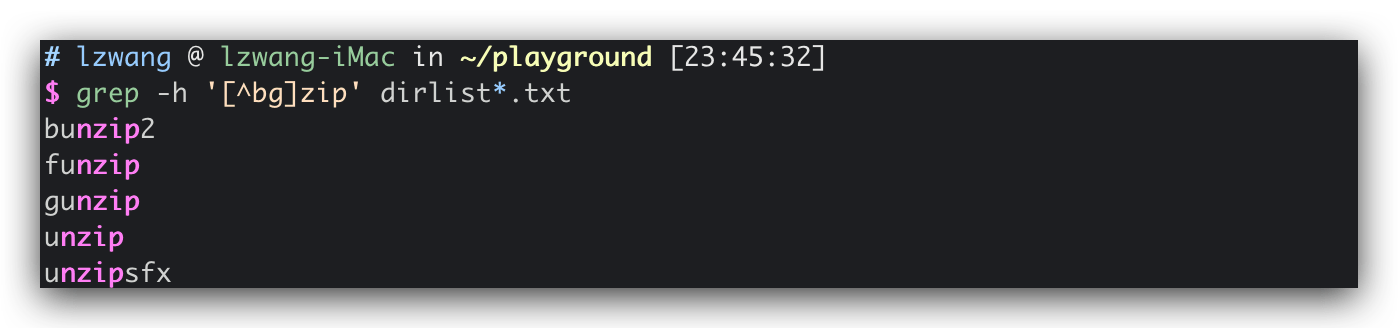3.3.3 字符范围匹配

 ``````1 `````` ``````grep -h '^[A-Z]' dirlist*.txt ``````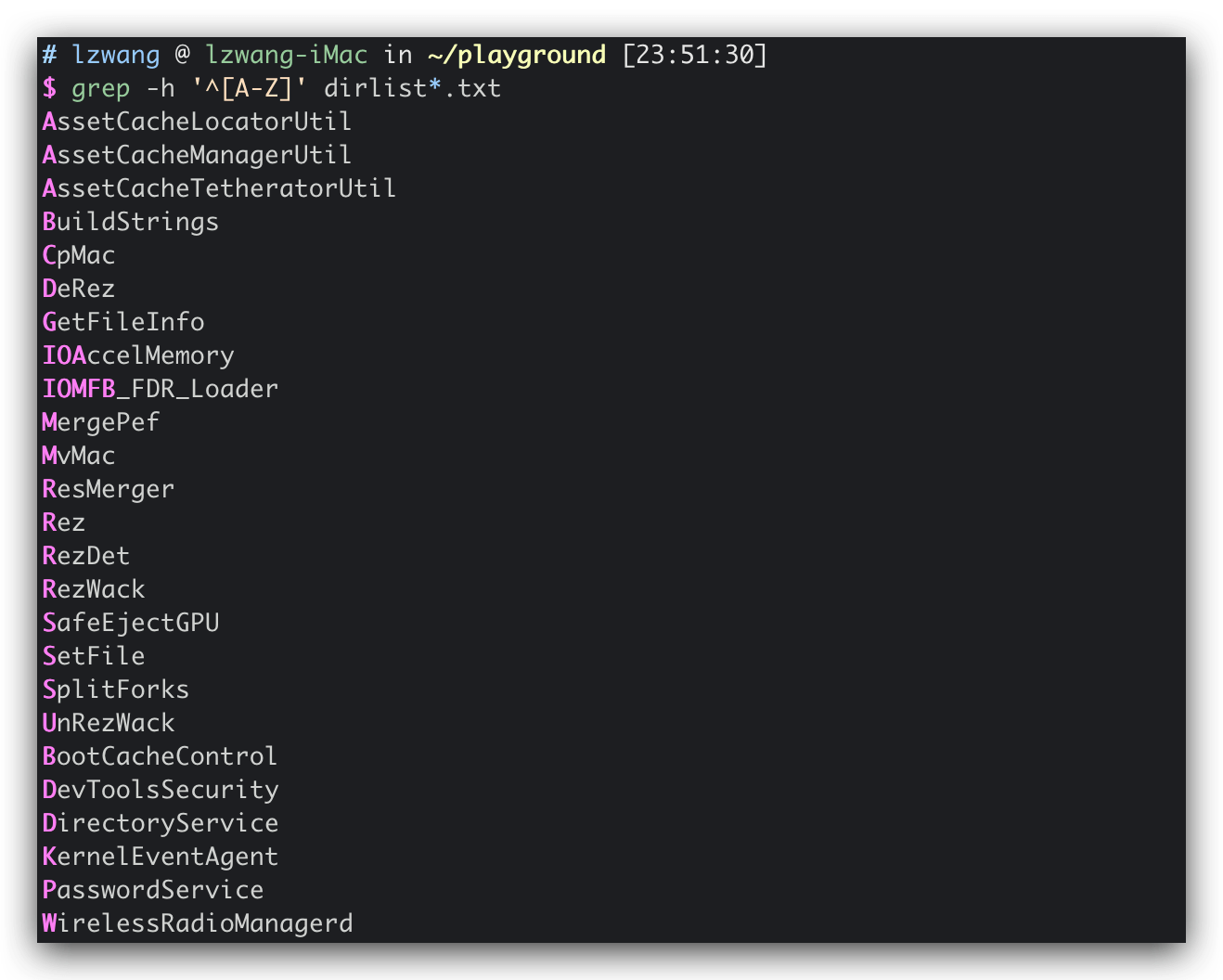``````1 `````` ``````grep -h '^[A-Za-z0-9]' dirlist*.txt ``````

 ``````1 `````` ``````grep -h '[-]' dirlist*.txt ``````

3.3.4 POSIX字符类

`[:alpha:]` 字母，即`[A-Za-z]`
`[:lower:]` 小写字母，即`[a-z]`
`[:upper:]` 大写字母，即`[A-Z]`
`[:digit:]` 数字，即`[0-9]`
`[:alnum:]` 字母和数字，即`[A-Za-z0-9]`
`[:xdigit:]` 16进制数字，即`[0-9A-Fa-f]`
`[:blank:]` 空格和制表符
`[:space:]` 空白字符，即ASCII的[`\t`, `\r`, `\n`, `\v`, `\f`]
`[:graph:]` 可见字符，即ASCII字符的 33～126
`[:cntrl:]` 控制字符，即ASCII字符的 0～31 和127
`[:pnuct:]` 标点符号，即ASCII的[ `, `~`, `!`, `@`, `#`, `\$`, `%`, `&`, `*`, `(`, `)`, `-`, `_`, `=`, `+`, `[`, `]`, `{`, `}`, `\`, `|`, `;`, `:`, `'`, `"`, `,` , `.`, `<`, `>`, `/`, `?`]
`[:print:]` 可打印字符，包括`[:graph:]`中的所有字符加上空格字符

## 4. 扩展正则表达式

BRE `^`, `\$`, `.`, `*`, `?`, `\`, `|`, `-`, `+`, `[`, `]`, `{`, `}`, `(`, `)`
ERE 除去BRE已有的外，还包括`(`, `)`, `{`, `}`, `?`, `+`, `|`（对应各自功能）

## 4.1 或（`|`）

4.1.1 基本使用

 ``````1 2 3 4 5 6 7 8 `````` ``````# BRE echo "AAA" | grep AAA # --> 匹配到 AAA echo "BBB" | grep BBB # --> 无匹配 # ERE echo "AAA" | grep -E 'AAA|BBB' # --> 匹配到 AAA echo "BBB" | grep -E 'AAA|BBB' # --> 匹配到 BBB echo "CCC" | grep -E 'AAA|BBB' # --> 无匹配 echo "CCC" | grep -E 'AAA|BBB|CCC' # --> 匹配到 CCC ``````

4.1.2 与其他符号结合使用

 ``````1 `````` ``````grep -Eh '^(bz|gz|zip)' dirlist*.txt ``````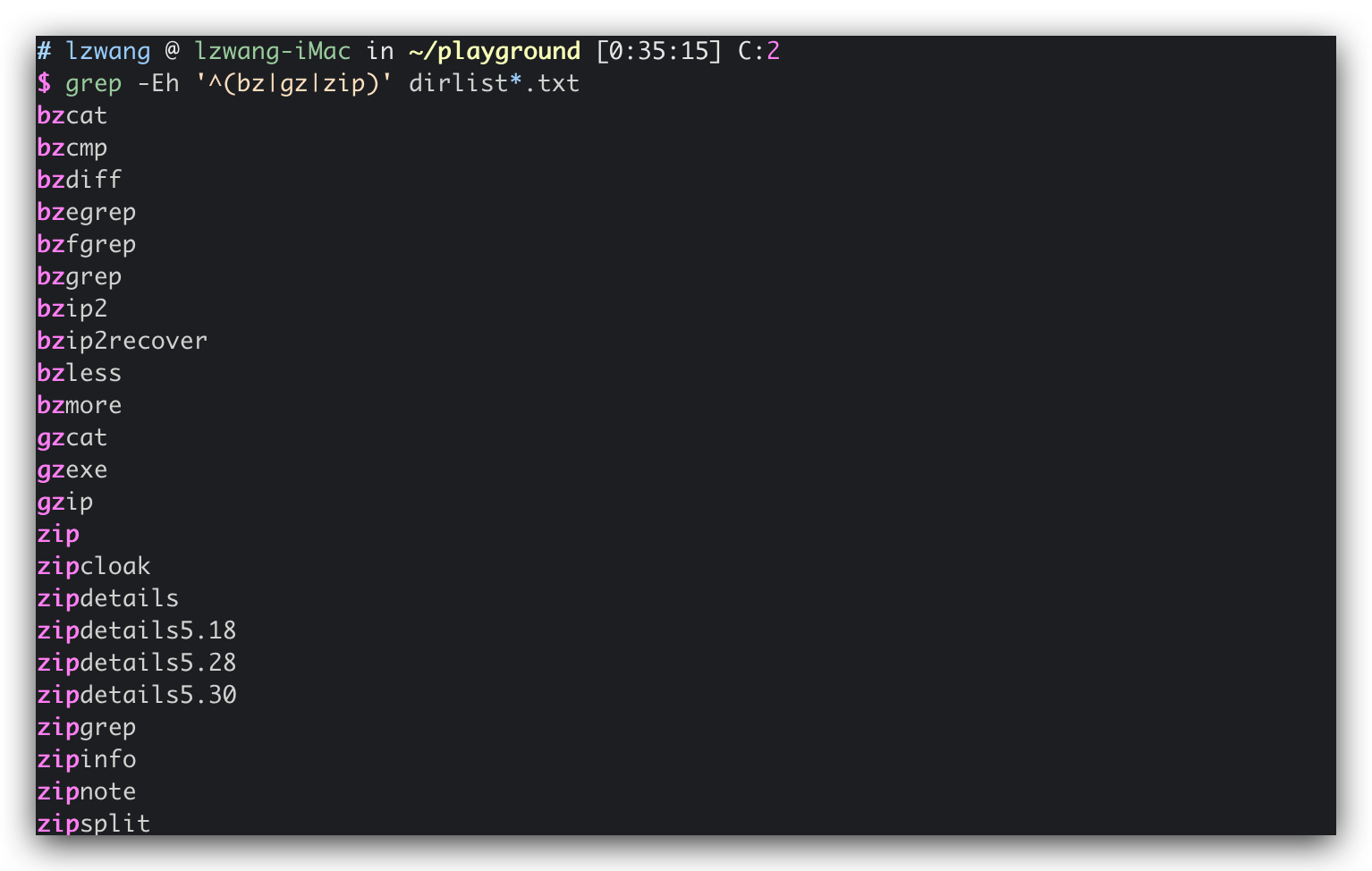``````1 `````` ``````grep -Eh '^bz|gz|zip' dirlist*.txt ``````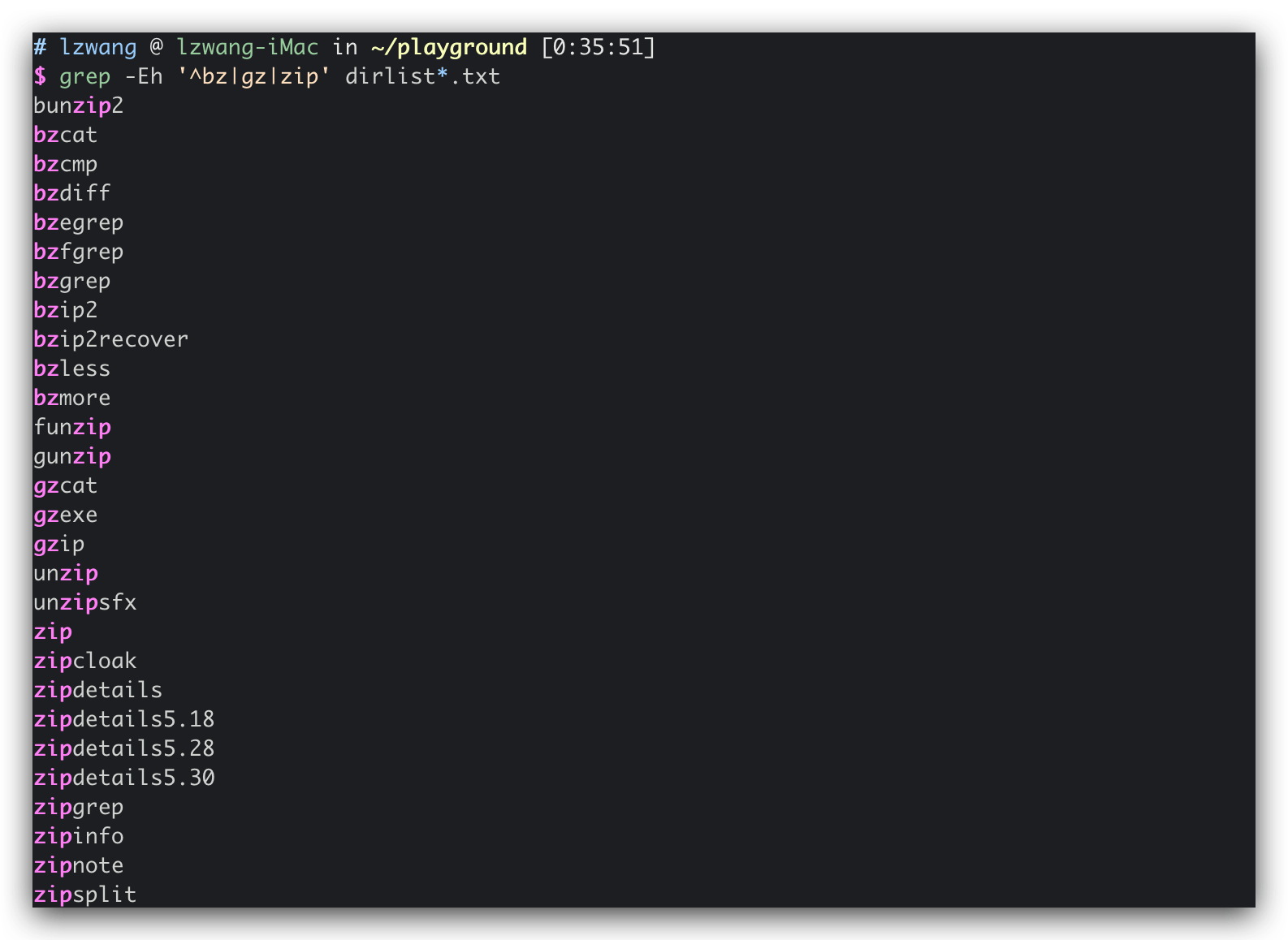## 4.2 匹配元素零次或一次（`?`）

`?`代表其前面的元素是可选的，匹配0或1次。

 ``````1 2 `````` ``````echo "(888) 123-4567" | grep -E '^\(?[0-9][0-9][0-9]\)? [0-9][0-9][0-9]-[0-9][0-9][0-9][0-9]\$' echo "888 123-4567" | grep -E '^\(?[0-9][0-9][0-9]\)? [0-9][0-9][0-9]-[0-9][0-9][0-9][0-9]\$' ``````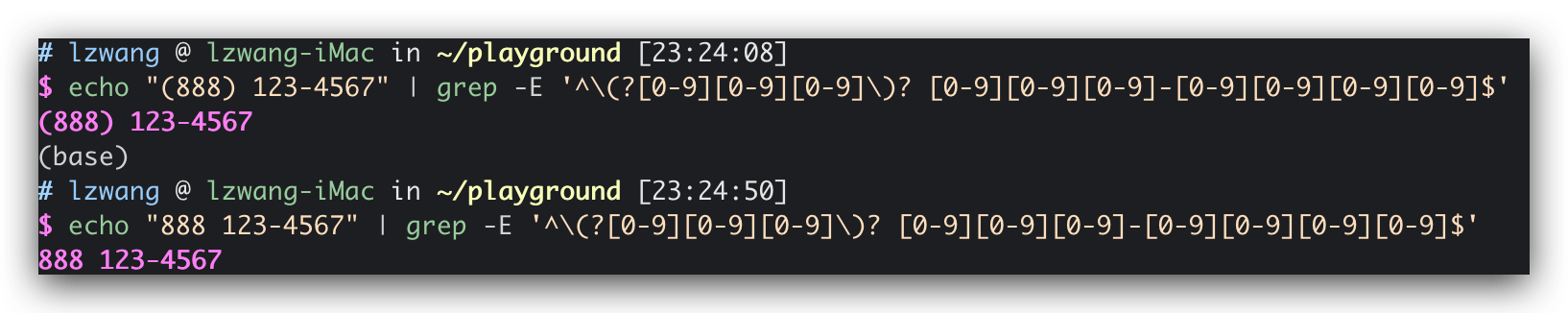## 4.3 匹配元素零次或多次（`*`）

 ``````1 2 3 `````` ``````echo "This works." | grep -E '[[:upper:]][[:upper:][:lower:] ]*\.' # 可以匹配 echo "This Works." | grep -E '[[:upper:]][[:upper:][:lower:] ]*\.' # 可以匹配 echo "this not works" | grep -E '[[:upper:]][[:upper:][:lower:] ]*\.' # 无法匹配 ``````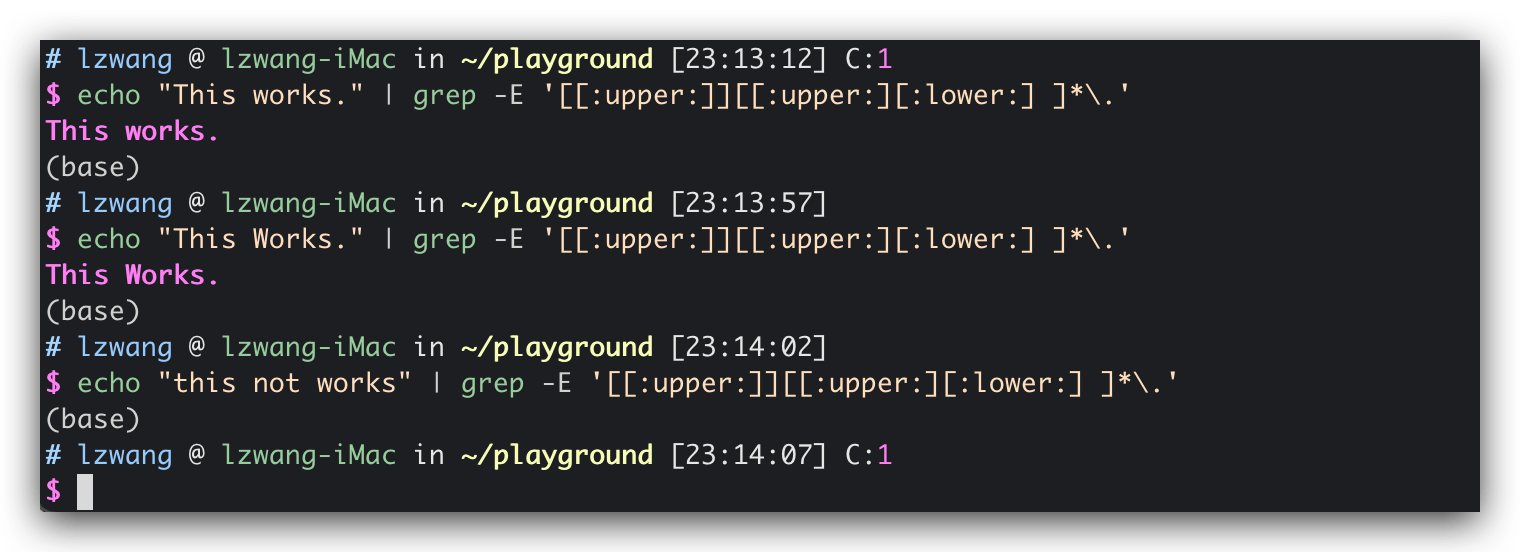## 4.4 匹配元素一次或多次（`+`）

`*`类似，但要求前面的字符至少出现一次。

 ``````1 2 3 4 `````` ``````echo "This that" | grep -E '^([[:alpha:]]+ ?)+\$' # 可以匹配 echo "a b c" | grep -E '^([[:alpha:]]+ ?)+\$' # 可以匹配 echo "a b 8" | grep -E '^([[:alpha:]]+ ?)+\$' # 无法匹配 echo "abc 8" | grep -E '^([[:alpha:]]+ ?)+\$' # 无法匹配 ``````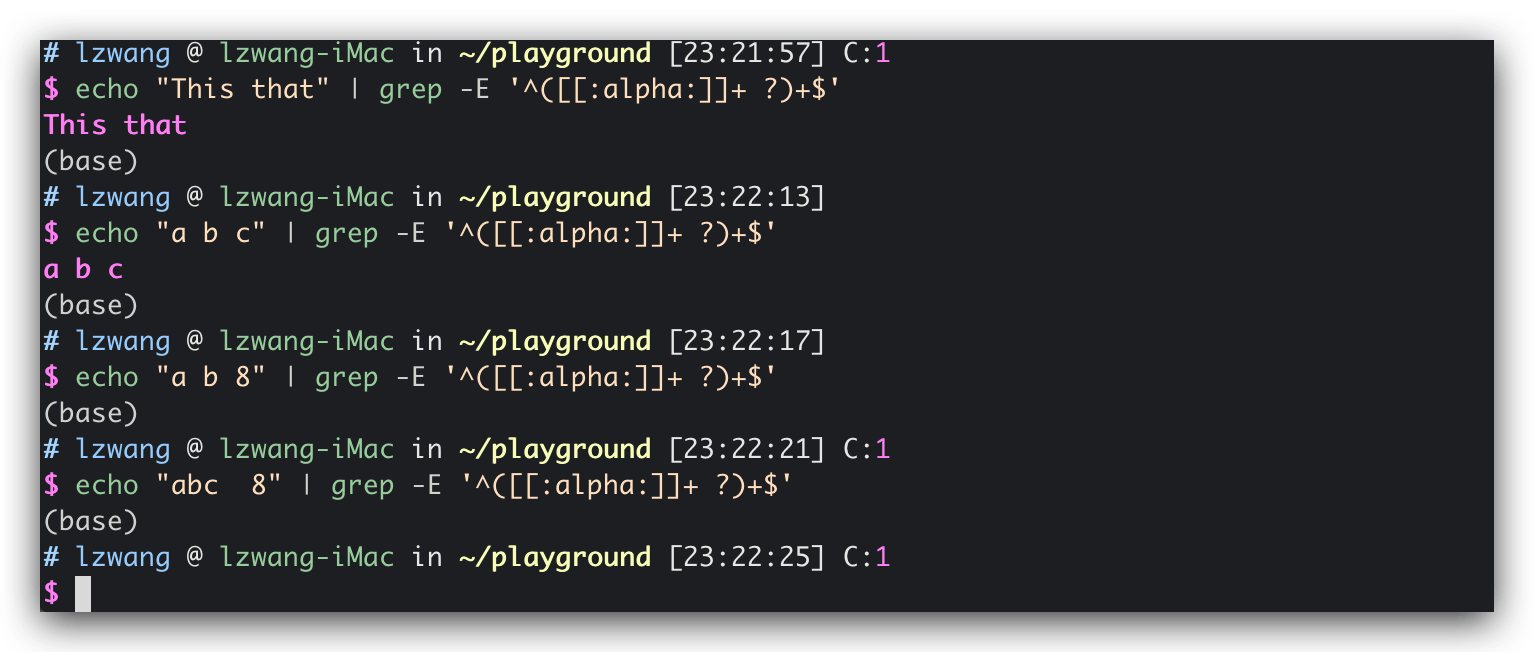## 4.5 匹配元素指定次数（`{}`）

1. `{n}`: 前面元素出现了`n`
2. `{n,m}`: 前面元素出现了`n~m`
3. `{n,}`: 前面元素出现了`>n`
4. `{,m}`: 前面元素出现了`<=m`

 ``````1 `````` ``````echo "(888) 123-4567" | grep -E '^\(?[0-9]{3}\)? [0-9]{3}-[0-9]{4}\$' ``````